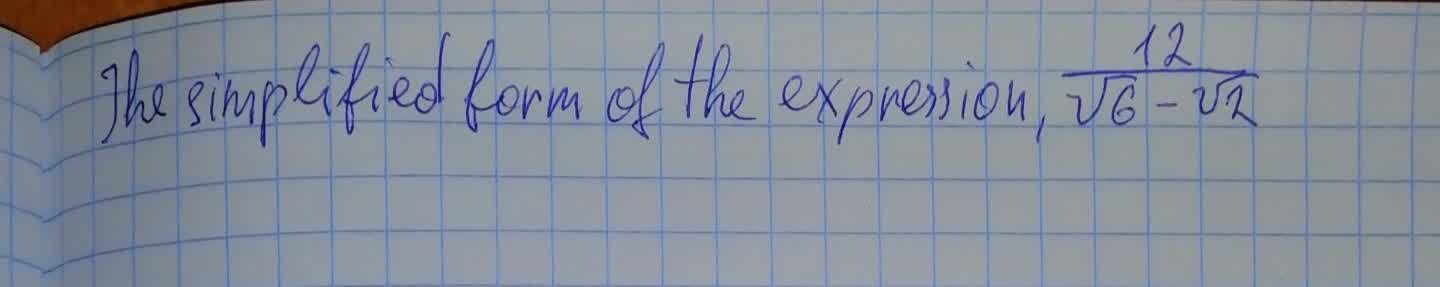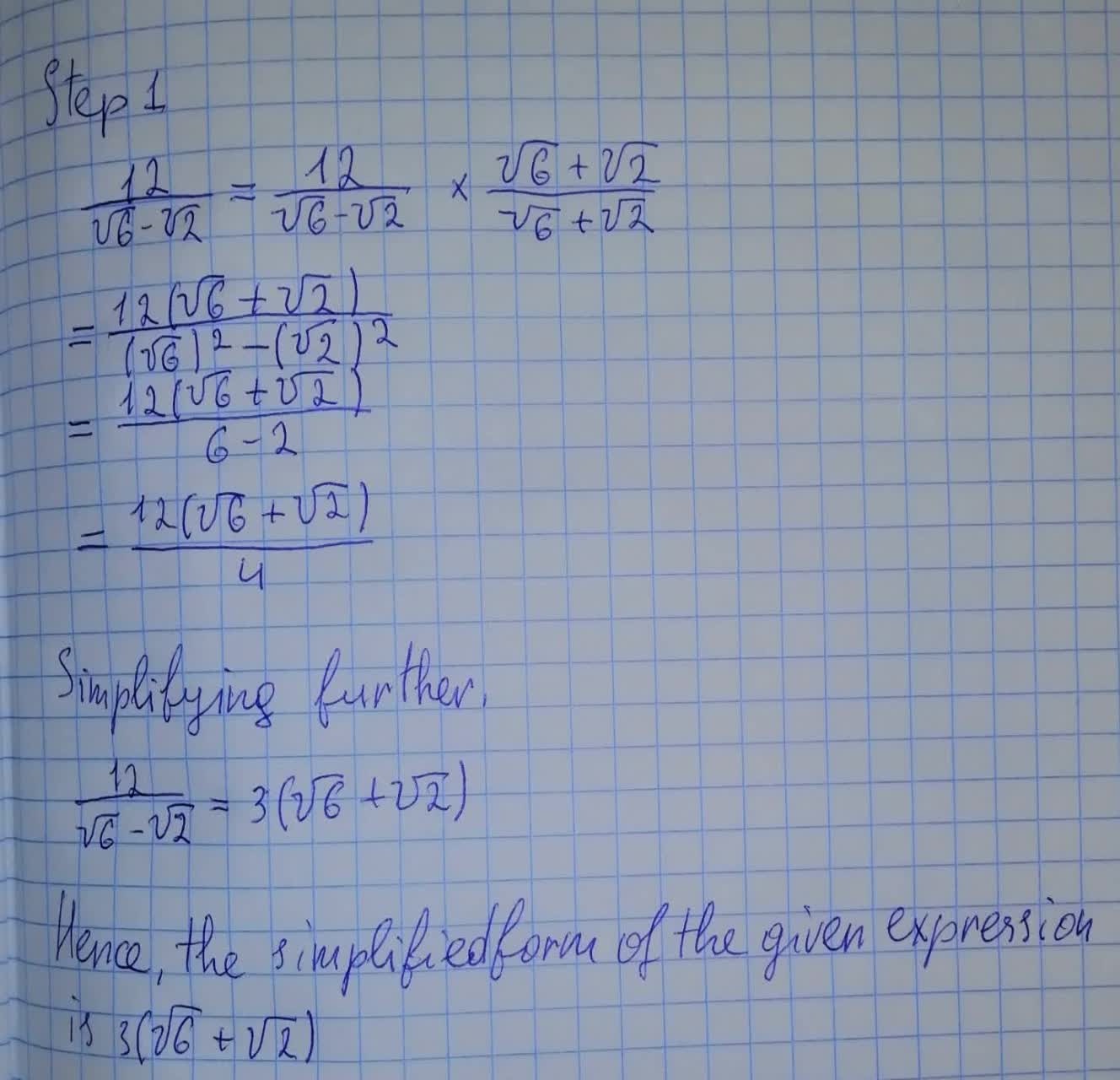Question# To calculate: The simplified form of the expression , \frac{12}{\sqrt{6}+\sqrt2}

Conic sections
ANSWEREDTo calculate: The simplified form of the expression , $$\displaystyle{\frac{{{12}}}{{\sqrt{{{6}}}+\sqrt{{2}}}}}$$